### 15年5700億，雙色球16227注一等獎數據中，我們發現了這些祕密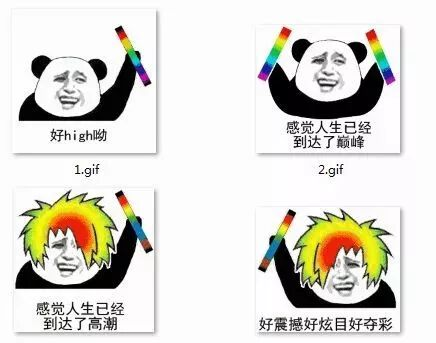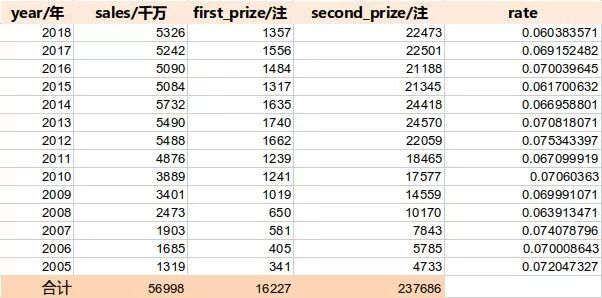▲2005-2018年，雙色球的數據統計

▲視頻來源：飛碟説

01 直方圖解讀歷年中獎注數

1. matplotlib.pyplot.bar(left, height, alpha=1, width=0.8, color=, edgecolor=, label=, lw=3)

• left：x軸的位置序列，一般採用arange函數產生一個序列；

• height：y軸的數值序列，也就是柱形圖的高度，一般就是我們需要展示的數據；

• alpha：透明度

• width：為柱形圖的寬度，一般這是為0.8即可；

• color或facecolor：柱形圖填充的顏色；

• edgecolor：圖形邊緣顏色

• label：解釋每個圖像代表的含義

• linewidth or linewidths or lw：邊緣or線的寬度

2. matplotlib.pyplot.legend()

plt.legend()函數主要的作用就是給圖加上圖例，plt.legend([x,y,z])裏面的參數使用的是list的的形式，默認情況下是將label值賦給它。

``import numpy as npimport matplotlib.pyplot as pltimport pandas as pddef DrawHistogram(read_name):    #讀取數據    read_name = r"C:\Users\Administrator\Desktop\ssq.csv"    fp = pd.read_csv(read_name)    first_prize = fp.first_prize    second_prize = fp.second_prize    #配置圖形參數    ind = np.arange(len(first_prize))    width = 0.5    fig, ax = plt.subplots()    rects1 = ax.bar(ind - width/2, first_prize, width, color='SkyBlue', label='First')    rects2 = ax.bar(ind + width/2, second_prize, width,color='IndianRed', label='Second')    ax.set_ylabel('Stakes')    ax.set_title('Stakes by year and rank')    plt.xticks(ind,(18,17,16,15,14,13,12,11,10,9,8,7,6,5))    ax.legend()     plt.show()if __name__=='__main__':   DrawHistogram(r"C:\Users\Administrator\Desktop\ssq.csv")``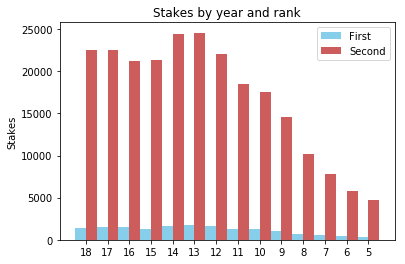▲歷年一等獎、二等獎中獎數

02 氣泡圖解讀銷售額的大小，與一等獎的佔比率並沒有關係

• scatter(x,y) 在向量 x 和 y 指定的位置創建一個包含圓形的散點圖。該類型的圖形也稱為氣泡圖。

• scatter(x,y,sz) 指定圓大小。要繪製大小相等的圓圈，請將 sz 指定為標量。要繪製大小不等的圓，請將 sz 指定為長度等於 x 和 y 的長度的向量。

• scatter(x,y,sz,c) 指定圓顏色。要以相同的顏色繪製所有圓圈，請將 c 指定為顏色名稱或 RGB 三元數。要使用不同的顏色，請將 c 指定為向量或由 RGB 三元數組成的三列矩陣。

``import pandas as pdimport numpy as npimport seaborn as snsimport matplotlib.pyplot as pltdef DrawBubble(read_name):    #讀取數據    fp = pd.read_csv(read_name)    x = fp.sales    y = fp.rate    z = fp.rate    #配置參數    sns.set(style = "whitegrid")    cm = plt.cm.get_cmap('RdYlBu')    fig,ax = plt.subplots(figsize = (12,10))    bubble = ax.scatter(x, y , s = (z - np.min(z) + 0.1) * 3000, c = z, cmap = cm, linewidth = 0.5, alpha = 0.5)    ax.grid()    fig.colorbar(bubble)     ax.set_xlabel('sales of year', fontsize = 15)    ax.set_ylabel('rate of something', fontsize = 15)    plt.show()if __name__=='__main__':    DrawBubble(r"C:\Users\Administrator\Desktop\ssq.csv")``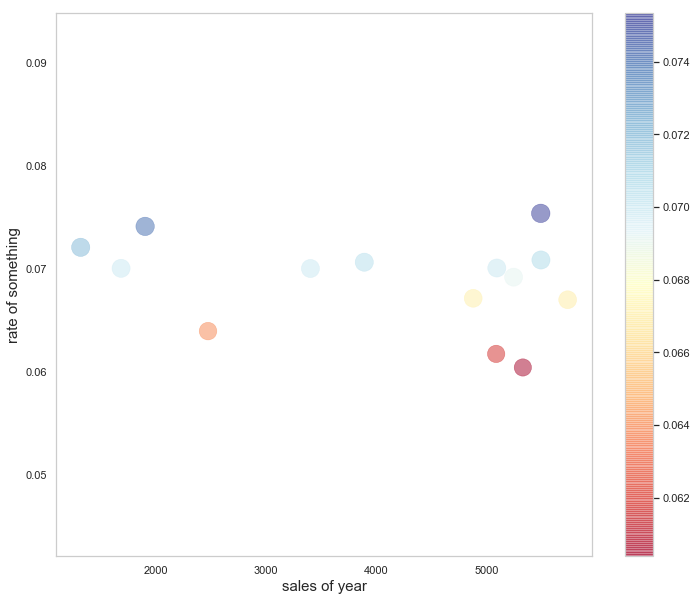▲歷年銷售額與一等獎的中獎佔比率之間關係

03 用Python畫一張好看的氣泡圖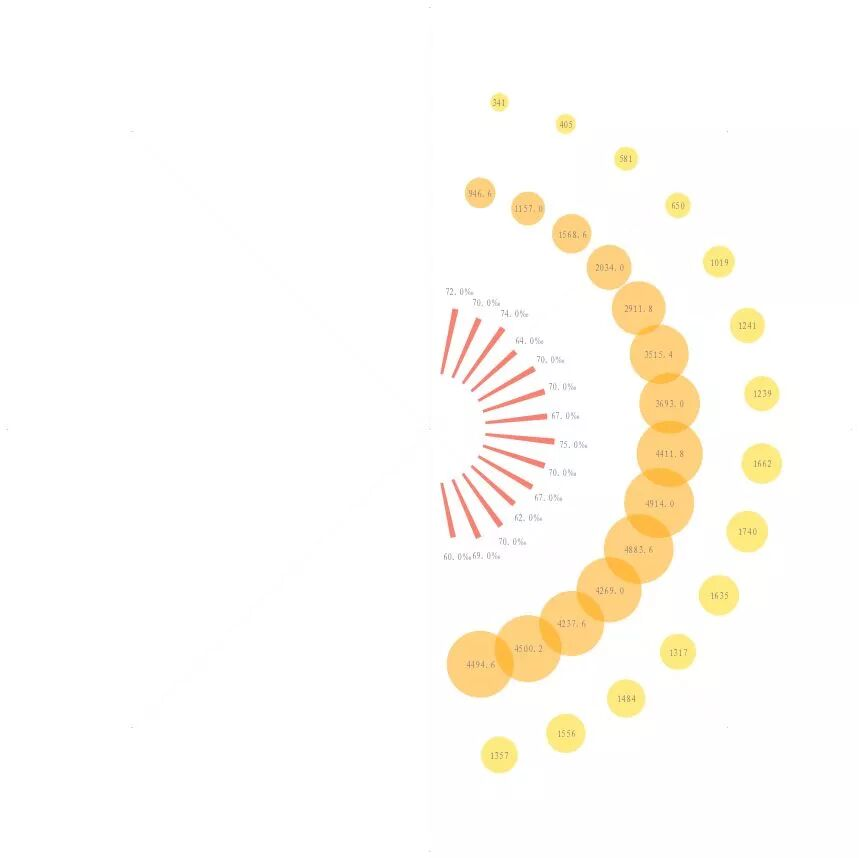``import numpy as npimport pandas as pdimport matplotlib.pyplot as pltimport matplotlib.font_manager#讀取數據read_name = r"C:\Users\Administrator\Desktop\ssq.csv"fp = pd.read_csv(read_name)first_prize = fp.first_prizesecond_prize = fp.second_prizerate = fp.rate#一等獎中獎注數S1=first_prizex1=[(3/2)*np.pi+np.pi/(len(S1)+1)*(i+1) for i in range(2*(len(S1)+1)) if i<len(S1)]y1=[180 for i in range(2*(len(S1)+1)) if i<len(S1) ]#為了畫出效果比例好看，將二等獎注數縮小5倍來表示S2=second_prize/5x2=[(3/2)*np.pi+np.pi/(len(S1)+1)*(i+1) for i in range(2*(len(S1)+1)) if i<len(S1)]y2=[130 for i in range(2*(len(S1)+1)) if i<len(S1) ]H1=[60/2,69/2,70/2,62/2,67/2,70/2,75/2,67/2,70/2,70/2,64/2,74/2,70/2,72/2]W1=[0.05 for i in range(2*(len(S1)+1)) if i<len(S1)]B=[30 for i in range(2*(len(S1)+1)) if i<len(S1)]fig=plt.figure(figsize=(13.44*2,7.5*2),facecolor='w')#建立一個座標系，projection='polar'表示極座標ax = fig.add_subplot(111,projection='polar',facecolor='w')ax.scatter(x=x1,y=y1,s=S1,color='Gold',alpha=0.5,linewidths=0)ax.scatter(x=x2,y=y2,s=S2,color='Orange',alpha=0.5,linewidths=0)ax.bar(x=x1, height=H1, width=W1,bottom=B,color='Salmon')plt.ylim(0,220)#去掉座標軸的為外面一層粗的線ax.spines['polar'].set_visible(False)plt.grid(color='gray', linestyle=':', linewidth=1,which='major',axis='x',alpha=0.1)ax.set_yticks([])ax.tick_params(axis='x',labelsize=0)font=matplotlib.font_manager.FontProperties(fname=r'E:\project\wordcloud\simfang.ttf')for i in range(len(x1)):    ax.text(x=x1[i],y=y1[i],s=S1[i],color='gray',ha='center', va= 'center',fontsize=12,fontproperties=font)for i in range(len(x1)):    ax.text(x=x1[i],y=y2[i],s=S2[i],color='gray',ha='center', va= 'center',fontsize=12,fontproperties=font)for i in range(len(x1)):    ax.text(x=x1[i],y=H1[i]+40,s="{}‰".format(H1[i]*2) ,color='gray',ha='center', va= 'center',fontsize=12,fontproperties=font)plt.show()fig.savefig('D:\\test.png',dpi=400,bbox_inches='tight',transparent=False)``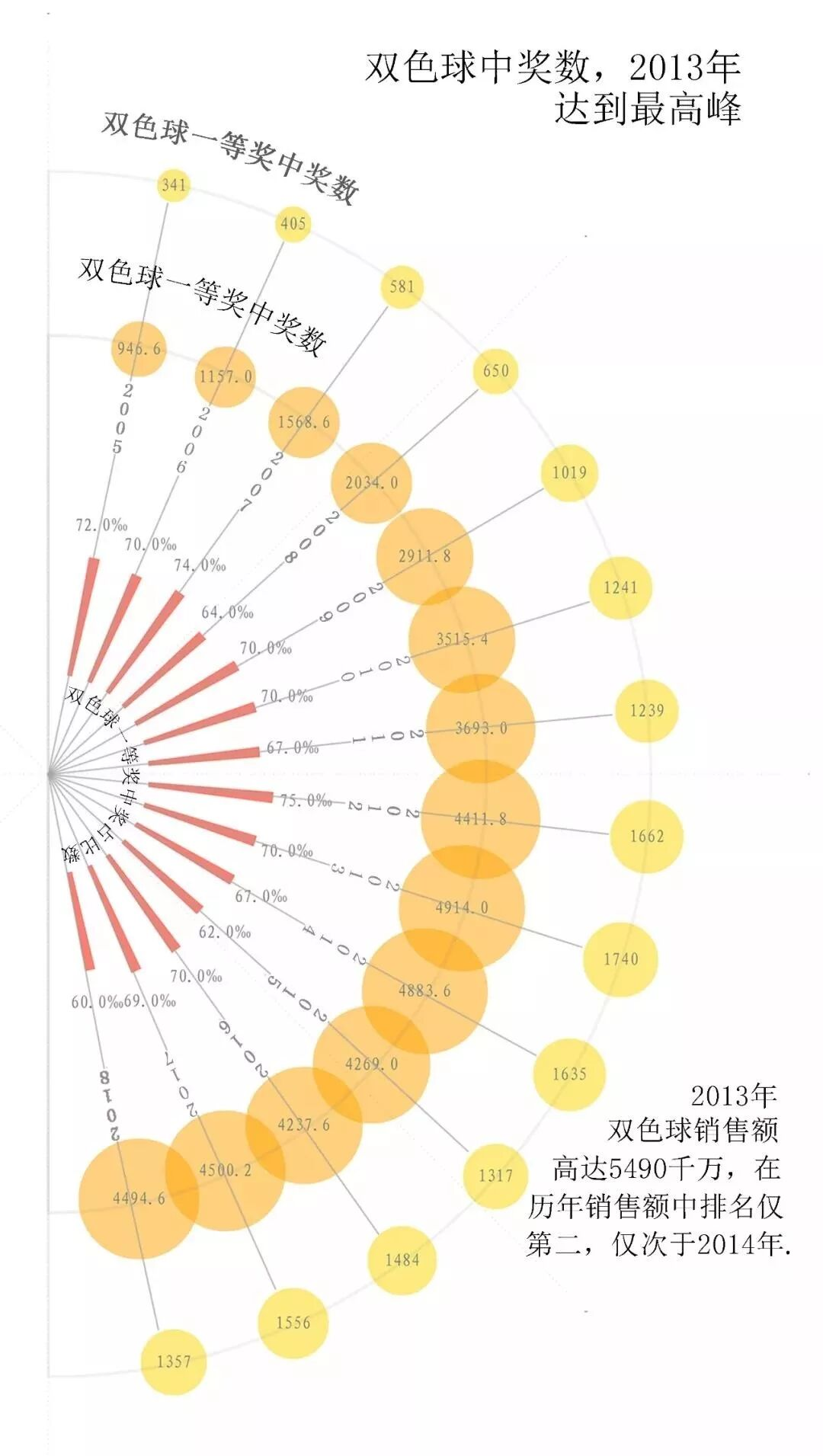▲雙色球一等獎中獎佔比率一直徘徊在7%左右

04 題外話：為什麼窮人更愛買彩票？

▲來源：飛碟説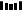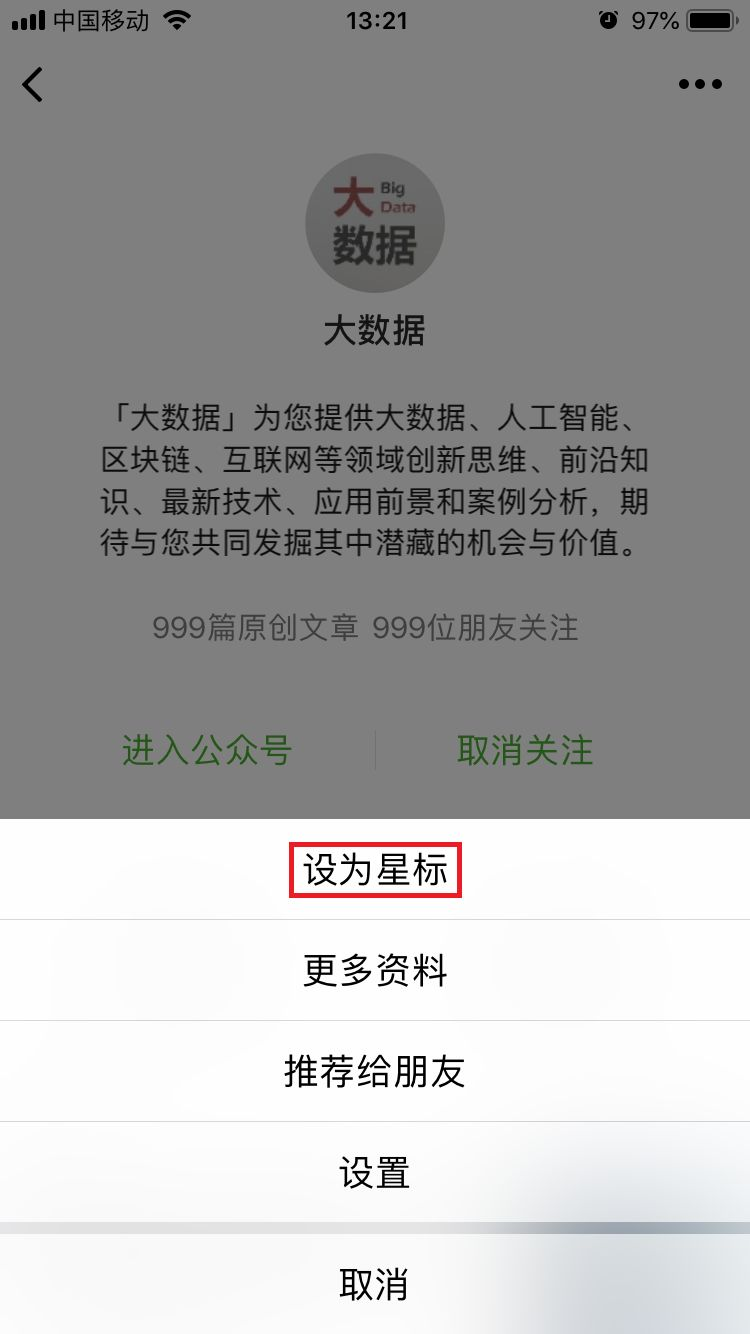PPT | 報吿 | 讀書 | 書單 | 乾貨

AI | 1024 | 段子 | 區塊鏈 | 數學

• 什麼樣的數據才有價值？應該怎樣收集和處理？終於有人講明白了

• 被兩會代表頻繁“點名”，2019的第一波風口要來了？

• 女神節，來聊聊這幾位神一般的“程序媛”

• 馬化騰提議加強基礎科學研究，中國“芯痛”能解決嗎？

Q: 你買彩票中過獎嗎？